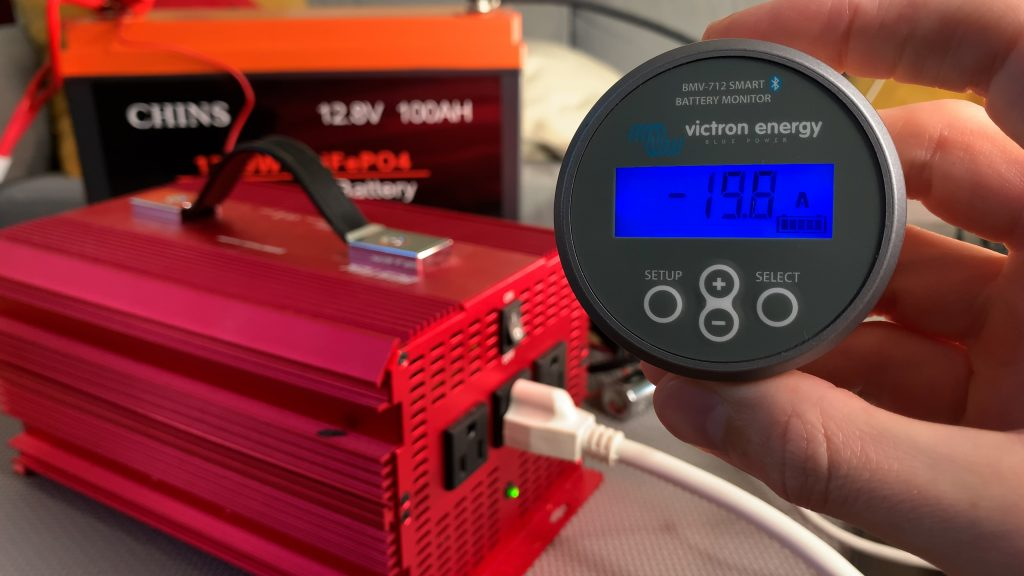# Amp Hours to Amps (Ah to A) Conversion Calculator

Use our calculator to easily convert amp hours to amps (Ah to A).

## Amp Hours to Amps Conversion Calculator

Error: This field is required.
Error: This field is required.
amps
Conversion formula: A = Ah ÷ hrs

Or would you rather convert amps to amp hours?

## Amp Hours to Amps Conversion Table

Here is a conversion table converting common values of amp hours to amps, over a duration of 1 hour and 1 day.

Note: Don't see the values you're looking for in this table? Use our Ah to A calculator at the top of this page to calculate them for your specific scenario.

## How to Convert Amp Hours to Amps (Ah to A)

To convert amp hours to amps, divide amp hours by hours. (If your time is in minutes or days, you'll need to first convert it to hours.)

Conversion formula: amps = amp hours ÷ hours

Abbreviated formula: A = Ah ÷ hrs

### Example

Let's say you have a 50Ah battery and you want to know how many amps it can provide over a 10 hour period.

You would calculate the amps by dividing the amp hours by the hours:

`50 Ah ÷ 10 hrs = 5 A`

So, your battery can provide 5 amps of current for 10 hours.

### Why Convert Amp Hours to Amps?If I want my 100Ah battery to run a device, such as this inverter, for around 5 hours, then the inverter should draw around 20 amps from the battery on average.

If you know how long you want a device to run and how many amp hours a battery has, you can calculate the maximum current draw in amps that the device should have.

Let's say you have a 100Ah LiFePO4 battery, and you want it to be able to power a device for 5 hours. To calculate the maximum current draw, you divide the battery's amp hours by the desired runtime in hours.

`100 Ah ÷ 5 hrs = 20 A`

So, if you want the battery to last for 5 hours, the device should draw around 20 amps on average.

Alternatively, you can convert amp hours to amps to estimate how much current you need to charge a battery at your desired rate.

For instance, let's say you have a 12V 50Ah LiFePO4 battery and you want to buy a 12V battery charger that can charge it in as little as 2 hours. How many amps should the charger be able to output?

`50 Ah ÷ 2 hrs = 25 A`

You need to buy a 12V battery charger capable of outputting up to 25 amps in order to meet your desired charge time.

Note: In reality, a battery's charge time depends on a number of factors such as battery type, depth of discharge, and the charger's charging algorithm. You can use our battery charge time calculator to get a more accurate estimate. Also, when using a battery, always try not to exceed the battery's max charging and discharging rates.

## How to Convert Amps to Amp Hours (A to Ah)

Need to convert the other way?

Amp hours are calculated by multiplying the number of amps by the number of hours.

Conversion formula: amp hours = amps × hours

Abbreviated formula: Ah = A × hrs

### Example

Let's say you have a small portable AC that draws 10 amps of current, and you use it for 3 hours per day. Here's how to calculate how many amp hours it consumes each day:

`10 A × 3 hrs = 30 Ah`

It uses 30 amp hours of electricity per day.

## More Electricity Calculators